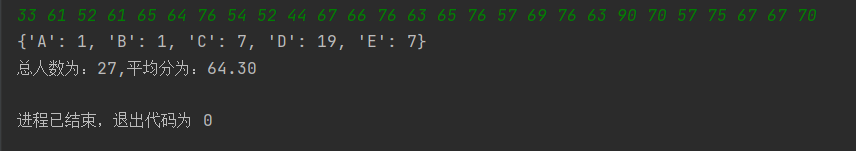2021-03-15 14:46

# Python利用字典进行成绩统计

分数             等级

[90, 100]     A

[80, 90)     B

[70, 80)     C

[60, 70)     D

[0, 60)     E

33 61 52 61 65 64 76 54 52 44 67 66 76 63 65 76 57 69 76 63 90 70 57 75 67 67 70

{'A': 1, 'B': 0, 'C': 7, 'D': 12, 'E': 7}

• 点赞
• 写回答
• 关注问题
• 收藏
• 邀请回答

#### 3条回答默认 最新

•多鱼的夏天 2021-03-15 15:32
已采纳
``````input = "33 61 52 61 65 64 76 54 52 44 67 66 76 63 65 76 57 69 76 63 90 70 57 75 67 67 70"
arr_input = map(int, input.split())
result = {'A': 0, 'B': 0, 'C': 0, 'D': 0, 'E': 0}
count = 0
total_score = 0
for x in arr_input:
count += 1
total_score += x
if x > 100:
continue
if x >= 90:
result['A'] += 1
elif x >= 80:
result['B'] += 1
elif x >= 70:
result['C'] += 1
elif x >= 60:
result['D'] += 1
else:
result['E'] += 1
print(result)
print('总人数为：%s，平均分为：%0.2f' % (count, total_score/count))
``````

点赞 1 评论
•coagenth 2021-03-15 15:46

``````
assert 0<=x<=100
if 90<=x<=100:
return 'A'
elif 80<=x<90:
return 'B'
elif 70 <= x < 80:
return 'C'
elif 60 <= x < 70:
return 'D'
else:
return 'E'
inp=[int(i) for i in input('students score:').split(' ')]
stud={}
for it in grds:
if it in stud:
stud[it]=stud.get(it,0)+1
else:
stud[it]=1
print(stud)
print('总人数为:',len(inp),',','平均分为:',round(sum(inp)/len(inp),2))``````
点赞 1 评论
•水星程序店 2021-03-15 15:36
``````def grade(str_grade: str):
testDic = {"A": 0, "B": 0, "C": 0, "D": 0, "E": 0}
for i in range(0, len(listStr) - 1):
if int(listStr[i]) >= 90:
testDic["A"] += 1
if int(listStr[i]) >= 80:
testDic["B"] += 1
if int(listStr[i]) >= 70:
testDic["C"] += 1
if int(listStr[i]) >= 60:
testDic["D"] += 1
else:
testDic["E"] += 1
print(testDic)
res = 0
for i in listStr:
res += int(i)
ag = res / len(listStr)
print("总人数为：" + str(len(listStr)) + ",平均分为：" + str('%.2f' % ag))

string_a = input()